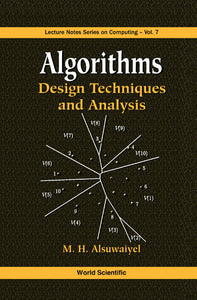# Algorithms: Design Techniques And Analysis

M H Alsuwaiyel

Format: Print Book

ISBN: 9789810237400

• SGD 222.56
Unit price per
Tax included.

Problem solving is an essential part of every scientific discipline. It has two components: (1) problem identification and formulation, and (2) solution of the formulated problem. One can solve a problem on its own using ad hoc techniques or follow those techniques that have produced efficient solutions to similar problems. This requires the understanding of various algorithm design techniques, how and when to use them to formulate solutions and the context appropriate for each of them. This book advocates the study of algorithm design techniques by presenting most of the useful algorithm design techniques and illustrating them through numerous examples.

Format: Hardcover
No of Pages: 544
Imprint: World Scientific
Publication date: 19990901
Series: Lecture Notes Series On Computing